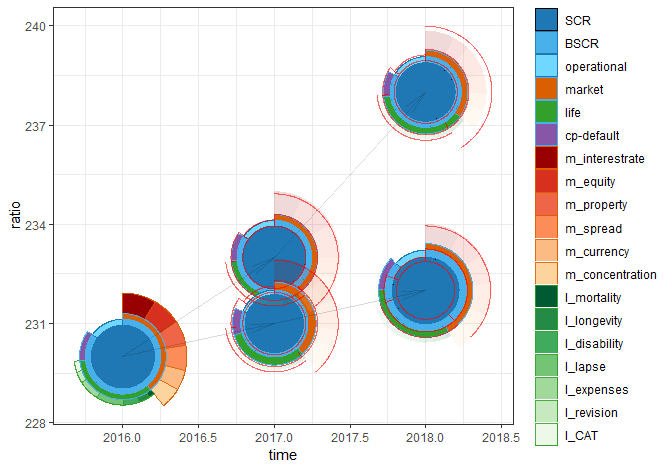# Showcase

#### 2019-01-03## Disclaimer

This documentation is in a rudimentary form for release 0.1.2. which is meant to see how much interest (not the financial one) this package generates.

## Vignettes

The following vignettes are available.

• ggsolvencyii
• plotdetails (placeholder)
• showcase (this vignette)
• coding_overview (placeholder)

On https://github.com/vanzanden/ggsolvencyii/tree/master/vignettes less rudimentary versions might be available between releases.

## Showcase

      ## the original dataset has three three-year scenarios,
## only two years of two branches are used here
testdata <- sii_z_ex1_data[sii_z_ex1_data$id <= 7,] testdata <- testdata[testdata$time <= 2018,]

## printing SCR values and interdependency of 'id' and 'comparewithid'
testdata[testdata$description == "SCR", ] #> time ratio description value id comparewithid #> 1 2016 230 SCR 23.00000 1 NA #> 2 2017 233 SCR 23.14993 2 1 #> 3 2018 238 SCR 19.99461 3 2 #> 5 2017 231 SCR 19.60600 5 1 #> 6 2018 232 SCR 25.74336 6 5 ## horizontalscaling to get round circles is depending on the dimensions of the canvas. ## automated scaling to canvas size is on the to do list. horizontalscaling = .22 ggplot2::ggplot() + ## a plain vanilla plot of one SCR buildup, the 'current situation': geom_sii_risksurface(data = testdata[testdata$id == 1, ],
mapping = ggplot2::aes(x = time,
y = ratio,
## x and y could for example be
## longitude and latitude
## in combination with plotted map
value = value,
id = id,
description = description,
fill = description, ## optional
color = description  ## optional
),
## all parameters are shown here,
## the values behind the outcommented are the default values
## how and what
## structure = sii_structure_sf16_eng,
## plotdetails = NULL,
## grouping
# levelmax = 99,
# aggregatesuffix = "other",
## scaling
## since the data in this geom is only a subset
## of 'testdata' manual scaling is needed
## each geom_sii_risksurface and geom_sii_riskoutline
## call returns the calculated (or given) maximum in an
## outputmessage
maxscrvalue =  25.7433642812936,
scalingx = horizontalscaling,
# scalingy = 1,
## rotation and squared
# rotationdegrees = NULL,
# rotationdescription = NULL,
# squared = FALSE,
## cosmetic
lwd = 0.25,
# alpha = 1
) +

ggplot2::theme_bw() +

## Combining several geom-calls might result in unexpected ordering of the legends
## It can help to plot the dataset which results in the most individual risks first.
ggplot2::scale_fill_manual(name = "risks", values = sii_z_ex1_fillcolors) +

ggplot2::scale_color_manual(name = "risks", values = sii_z_ex1_edgecolors) +

## a second instance of geom_sii_risksurface, all data (1+2*2 id's) is used
## by using a plotdetails dataframe not all calculated circle segments are plotted
geom_sii_risksurface(data = testdata,
mapping = aes(x = time,y = ratio,value = value,  id = id,
description = description,
fill = description, color = description
),
## two plotdetailstables are used for this showcase:
## this one indicates that only levels 1-3 are plotted in geom_sii_risksurface
## sii_z_ex1_plotdetails2 indicates only levels 4.xx and 5.xx are plotted
## this dataset has only 4.01, 4.02 levels present (market- and life subrisks )
plotdetails = sii_z_ex1_plotdetails,
scalingx = horizontalscaling,
lwd = 0.25,
alpha = 1.0
) +

## this third instance of geom_sii_risksurface plots only the levels 4.01 and 4.02
## by using the other plotdetails dataframe.
## A small alpha has the effect that these levels are less obtrusive,
## giving a overview of SCR results, but still showing all information
## 'color is NA', in the options means it does only plot the surface of the polygons.
## (geom_polygon is the basis for the actual plotting),
geom_sii_risksurface(data = testdata,
mapping = aes(x = time, y = ratio, value = value,  id = id,
description = description,
fill = description #,
## outcommenting here is not enough to prevent
## outlines to be plotted ...
# color = description
),
plotdetails = sii_z_ex1_plotdetails2,
scalingx = horizontalscaling,
alpha = 0.15,
## ... explicit no (edge)coloring is neccesary
color = NA
) +
## Arrows are plotted to connect 'id' and 'comparewithid' combinations.
## This helps in understanding the outlines of the following geom_sii_riskoutline call
geom_sii_riskconnection(data = testdata,
mapping = aes(x = time, y = ratio, id = id,
## for geom_sii_riskconnection comparewithid is a required aesthetic.
## (this is is not the case for geom_sii_riskoutline)
comparewithid = comparewithid
),
arrow = ggplot2::arrow(angle = 10, type = "open" ),
alpha = 0.15
) +

## geoms_sii_riskoutline uses other columns in plotdetails than geoms_sii_risksurface
## for each line segment for each defined description or level plotting can be
## switched on or off.
## a risk-partition (apart from the full circle SCR) has four outline segments.
## two radii and an inner and outer circle segment.
## sii_z_ex1_plotdetails defines only the outer circle segments ('outline2') to be plotted
## for levels 1, 4.01 and 4.02 AND for the indivual risks operational and cp-default.
## these individual risks are on level 2 and 3 but have no subrisks.
geom_sii_riskoutline(data = testdata,
mapping = aes(x = time, y = ratio, value = value,  id = id,
description = description,
comparewithid = comparewithid,
),
## only sii_z_ex1_plotdetails is used with the outline-geom.
plotdetails = sii_z_ex1_plotdetails,
scalingx = horizontalscaling,
color = "red",
lwd = 0.25,
alpha = 0.6,
)
#> scaling is based on inputvalue (maxscrvalue) of 25.7433642812936
#> scaling is based on a max (level= 1) value of 25.7433642812936
#> scaling is based on a max (level= 1) value of 25.7433642812936
#> scaling is based on a max (level= 1) value of 25.7433642812936## cleanup ============================================================== =====
rm(testdata) ; rm(horizontalscaling)
## ====================================================================== =====